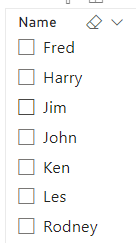cancel
Showing results for
Did you mean:Helper II

## Count for all sections of courses completed

Hi,

Need assistance with the following

3 tables Names, Courses and Data.

Data contains all records of courses completed for each Person

Courses contains grouping of Skills completed. If Fred has completed Course A, B and C he has then completed Skill S1. If Fred has also completed Coureses D and E but not F Skill S2 is not completed.

I got a part the way there with the following Calculated Column. This only works if they only complete one Skill set. If they complete extra courses this does not work. As you can see for Jim

All Complete =
VAR __Table = SUMMARIZE(FILTER('Data',[Name]=EARLIER([Name])),[Skill])
RETURN
SWITCH(
TRUE(),
MAXX(__Table,[Skill])="S1" && COUNTROWS(__Table)=1, "S1 Completed",
MAXX(__Table,[Skill])="S2" && COUNTROWS(__Table)=1, "S2 Completed",
MAXX(__Table,[Skill])="S3" && COUNTROWS(__Table)=1, "S3 Completed",
MAXX(__Table,[Skill])="S4" && COUNTROWS(__Table)=1, "S4 Completed",
MAXX(__Table,[Skill])="S5" && COUNTROWS(__Table)=1, "S5 Completed",
MAXX(__Table,[Skill])="S6" && COUNTROWS(__Table)=1, "S6 Completed",
"Not Completed"
)1 ACCEPTED SOLUTIONSuper User

@zenton , A new measure

new measure =
var _count = calculate(Countrows(Summarize(Data, Courses[Skill])), allexcept(Data,Names[Name],Courses[Skill]))
var _all calculate(Countrows(Summarize(Courses, Courses[Skill])), allexcept(Courses,Courses[Skill]))
return
if(_count = _all , max(Courses[Skill]) & " is completed" , "Not completed")

!! Microsoft Fabric !!
Microsoft Power BI Learning Resources, 2023 !!
Learn Power BI - Full Course with Dec-2022, with Window, Index, Offset, 100+ Topics !!
Did I answer your question? Mark my post as a solution! Appreciate your Kudos !! Proud to be a Super User! !!Super User

@zenton , A new measure

new measure =
var _count = calculate(Countrows(Summarize(Data, Courses[Skill])), allexcept(Data,Names[Name],Courses[Skill]))
var _all calculate(Countrows(Summarize(Courses, Courses[Skill])), allexcept(Courses,Courses[Skill]))
return
if(_count = _all , max(Courses[Skill]) & " is completed" , "Not completed")

!! Microsoft Fabric !!
Microsoft Power BI Learning Resources, 2023 !!
Learn Power BI - Full Course with Dec-2022, with Window, Index, Offset, 100+ Topics !!
Did I answer your question? Mark my post as a solution! Appreciate your Kudos !! Proud to be a Super User! !!